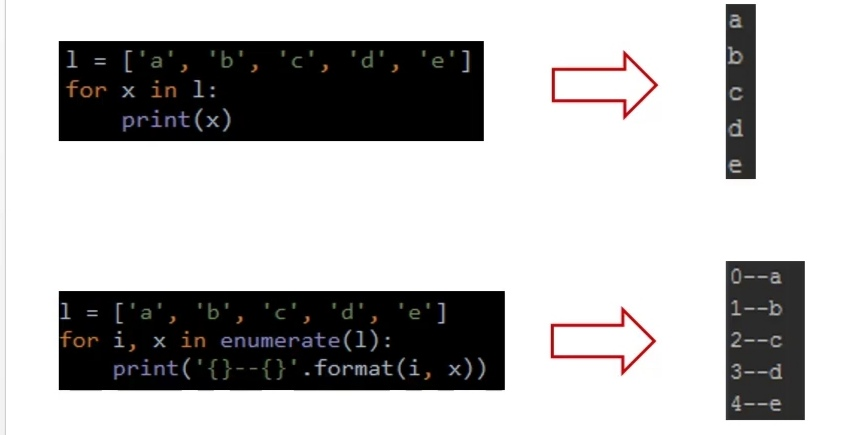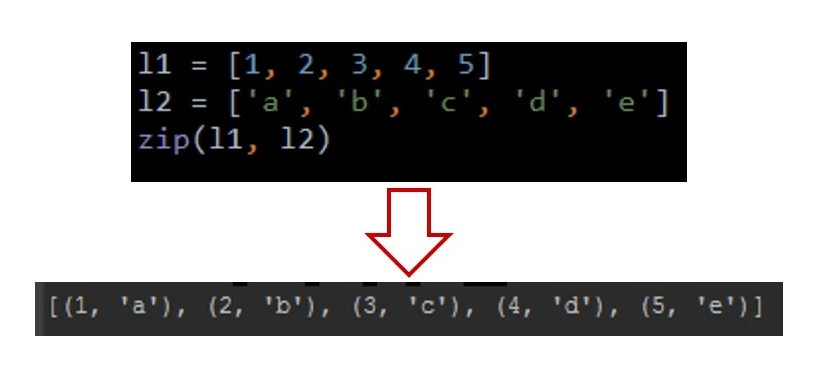## 模拟掷两个色子代码：

``````import random as t
def roll_dice():
roll = t.randint(1,6)
return roll

def main():
"""
主函数
"""
total_times = 1000000
#初始化列表[0,0,0,0,0,0,0,0,0,0,0]
list1 =  * 11
#初始化点数列表
roll_list = list(range(2,13))
roll_dict = dict(zip(roll_list,list1))
for i in range(total_times):
roll1 = roll_dice()
roll2 = roll_dice()
for j in range(2,13):
if (roll1 + roll2) == j:
roll_dict[j] += 1
for i, result in roll_dict.items():
print("点数{}的次数：{},频率{}".format(i,result,result / total_times))
if __name__ == "__main__":
main()

``````

``````点数2的次数：27662,频率0.027662

``````

## 数据可视化

``````import random as t
import matplotlib.pyplot as plt
def roll_dice():
roll = t.randint(1,6)
return roll

def main():
"""
主函数
"""
total_times = 100
#初始化列表[0,0,0,0,0,0,0,0,0,0,0]
list1 =  * 11
#初始化代数列表
roll_list = list(range(2,13))
roll_dict = dict(zip(roll_list,list1))
#记录色子的结果
roll1_list = []
roll2_list = []
for i in range(total_times):
roll1 = roll_dice()
roll2 = roll_dice()
roll1_list.append(roll1)
roll2_list.append(roll2)
for j in range(2,13):
if (roll1 + roll2) == j:
roll_dict[j] += 1
for i, result in roll_dict.items():
print("点数{}的次数：{},频率{}".format(i,result,result / total_times))
#数据可视化
x = range(1,total_times + 1)
plt.scatter(x,roll1_list,c = "red",alpha = 0.5)
plt.scatter(x,roll2_list,c= "green",alpha= 0.5)
plt.show()
if __name__ == "__main__":
main()

``````

## 数据可视化结果：• enumerate()函数用于将可遍历的列表转换为一个索引序列,一般用于for循环中，同时列出元素和元素的索引号。转换字典用 items() 函数。• zip()函数用于将对应的元素打包成一个元组。dic(zip(l1 + l2))

### TD18系列多功能精密校准器厂家校准仪器_御前的博客-程序员秘密

TD18系列是一款宽范围、高精度的多功能校准器，内置超精密的交直流电压源和电流源，可输出性能优异的直流电压、直流电流、直流功率及交流电压、交流电流、交流功率、相位、频率等电参量。

### socket技术详解（看清socket编程）_weixin_30828379的博客-程序员秘密

https://blog.csdn.net/weixin_39634961/article/details/80236161socket编程是网络常用的编程，我们通过在网络中创建socket关键字来实现网络间的通信，通过收集大量的资料，通过这一章节，充分的了解socket编程，文章用引用了大量大神的分析，加上自己的理解，做个总结性的文章1：socket大致介绍 socket编...